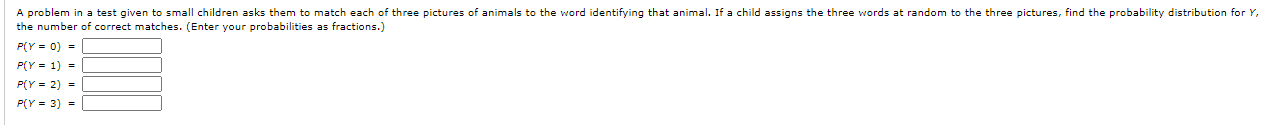# Question Solved1 AnswerA problem in a test given to small children asks them to match each of three pictures of animals to the word identifying that animal. If a child assigns the three words at random to the three pictures, find the probability distribution for Y, the number of correct matches. (Enter your probabilities as fractions.) PlY = 0) = PIY = 1) = PLY = 2) = PlY = 3) =Transcribed Image Text: A problem in a test given to small children asks them to match each of three pictures of animals to the word identifying that animal. If a child assigns the three words at random to the three pictures, find the probability distribution for Y, the number of correct matches. (Enter your probabilities as fractions.) PlY = 0) = PIY = 1) = PLY = 2) = PlY = 3) =
More
Transcribed Image Text: A problem in a test given to small children asks them to match each of three pictures of animals to the word identifying that animal. If a child assigns the three words at random to the three pictures, find the probability distribution for Y, the number of correct matches. (Enter your probabilities as fractions.) PlY = 0) = PIY = 1) = PLY = 2) = PlY = 3) =